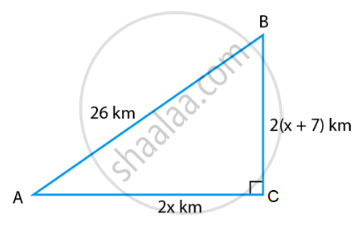# For going to a city B from city A, there is a route via city C such that AC ⊥ CB, AC = 2x km and CB = 2(x + 7) km. It is proposed to construct a 26 km highway which directly connects - Mathematics

Sum

For going to a city B from city A, there is a route via city C such that AC ⊥ CB, AC = 2x km and CB = 2(x + 7) km. It is proposed to construct a 26 km highway which directly connects the two cities A and B. Find how much distance will be saved in reaching city B from city A after the construction of the highway.

#### Solution

Given,

AC ⊥ CB,

AC = 2x km,

CB = 2(x + 7) km and AB = 26 km

Thus, we get ∆ACB right angled at C.

Now, from ∆ACB,

Using Pythagoras Theorem,

AB2 = AC2 + BC2

⇒ (26)2 = (2x)2 + {2(x + 7)}2

⇒ 676 = 4x2 + 4(x2 + 196 + 14x)

⇒ 676 = 4x2 + 4x2 + 196 + 56x

⇒ 676 = 82 + 56x + 196

⇒ 8x2 + 56x – 480 = 0Dividing the equation by 8, we get,

x2 + 7x – 60 = 0

x2 + 12x – 5x – 60 = 0

x(x + 12) – 5(x + 12) = 0

(x + 12)(x – 5) = 0

∴ x = – 12 or x = 5

Since the distance can’t be negative, we neglect x = –12

∴ x = 5

Now,

AC = 2x = 10km

BC = 2(x + 7) = 2(5 + 7) = 24 km

Thus, the distance covered to city B from city A via city C = AC + BC

AC + BC = 10 + 24

= 34 km

Distance covered to city B from city A after the highway was constructed = BA = 26 km

Therefore, the distance saved = 34 – 26 = 8 km.

Concept: Application of Pythagoras Theorem in Acute Angle and Obtuse Angle
Is there an error in this question or solution?

#### APPEARS IN

NCERT Mathematics Exemplar Class 10
Chapter 6 Triangles
Exercise 6.4 | Q 6 | Page 73

Share